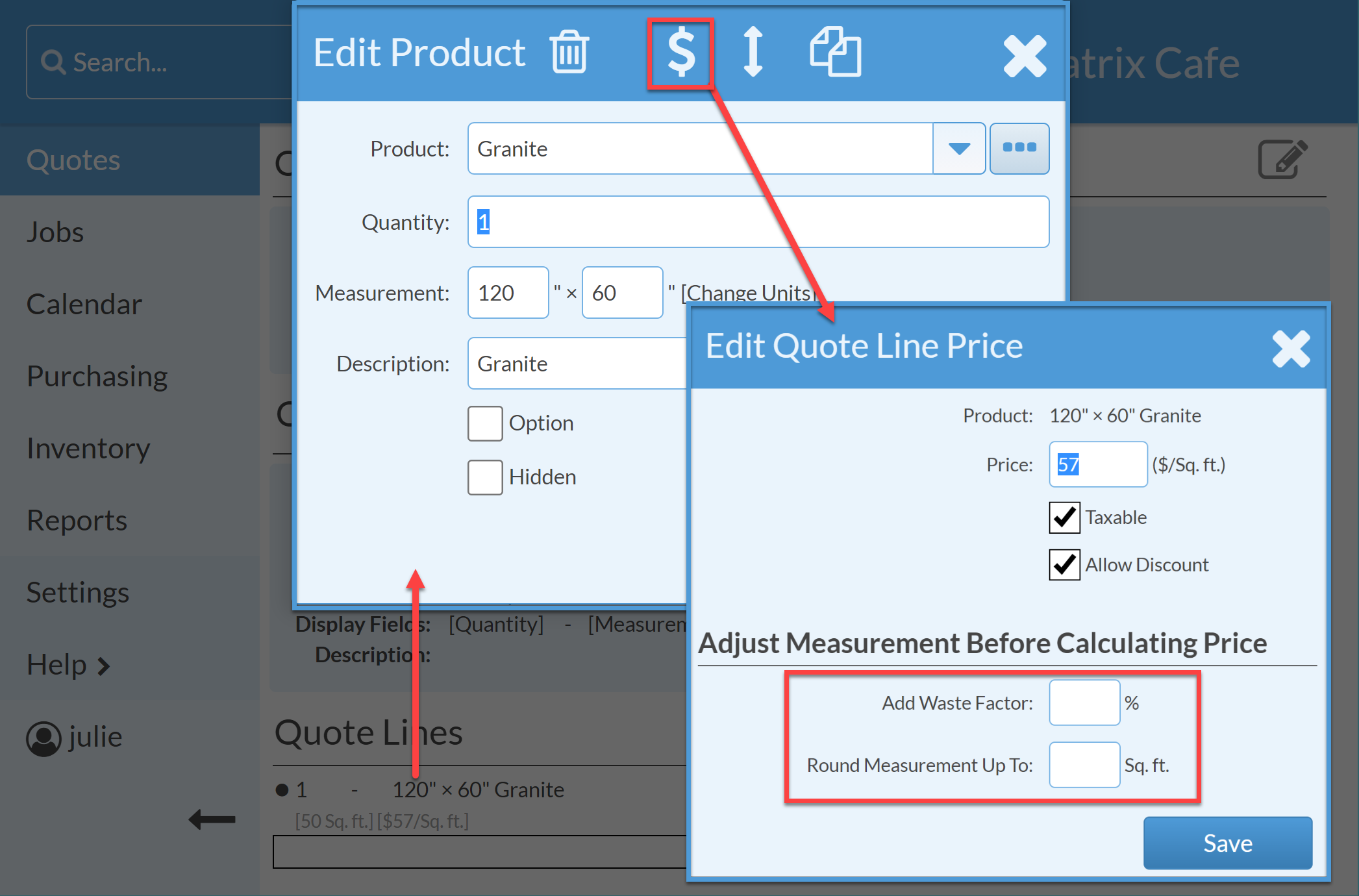Add a Waste Factor and/or round a measurement up before the price on a Quote is calculated to ensure prices are not underbid.

Either a Waste Factor % can be added, or you can Round Measurement Up To. The calculated measurements will be adjusted by these amounts in order to calculate the price. If you enter both fields, the percentage for Waste Factor will be applied before rounding.

1. Open a Quote > Click on the Product in Quote Lines.
2. Click on Edit Price icon.
3. Add either a Waste Factor, a Rounding Measurement, or both.EXAMPLE 1
Measurement = 50 sq. ft.
Waste Factor % = 10%
Round Measurement Up To: ___
Measurement used in pricing = 55 sq. ft.

EXAMPLE 2
Measurement = 51 sq. ft.
Waste Factor % ___
Round Measurement Up To: 50 sq. ft.
Measurement used in pricing = 100 sq. ft.

EXAMPLE 3
Measurement = 50 sq. ft.
Waste Factor % = 10%
Round Measurement Up To: 50 sq. ft.

Measurement used in pricing = 100 sq. ft.